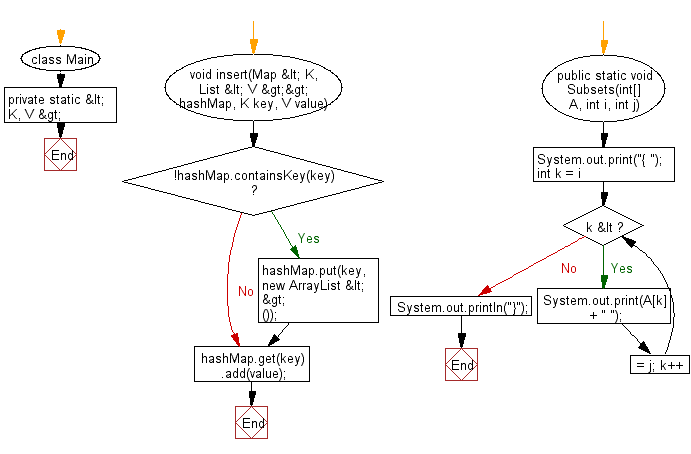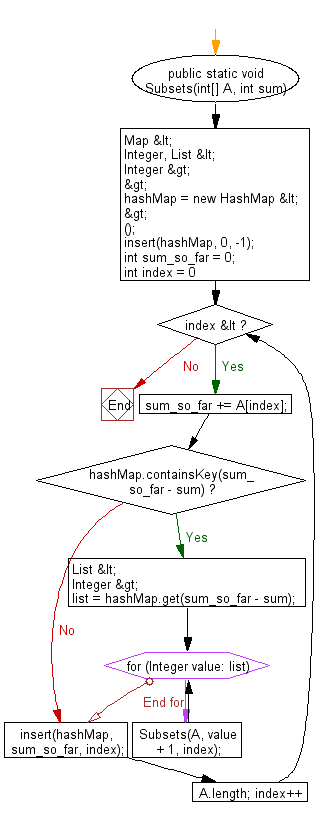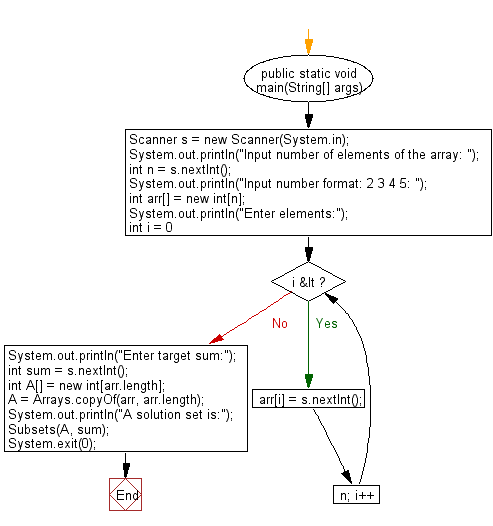# Java Exercises: Find all unique combinations from a collection of candidate numbers

## Java Basic: Exercise-209 with Solution

Write a Java program to find all unique combinations from a collection of candidate numbers. The sum of the numbers will be equal to a given target number.

Sample Solution:

Java Code:

``````import java.util.*;
class Main {
private static < K, V > void insert(Map < K, List < V >> hashMap, K key, V value) {
if (!hashMap.containsKey(key)) {
hashMap.put(key, new ArrayList < > ());
}
}
public static void Subsets(int[] A, int i, int j) {
System.out.print("{ ");
for (int k = i; k <= j; k++) {
System.out.print(A[k] + " ");
}
System.out.println("}");
}

public static void Subsets(int[] A, int sum) {
Map < Integer, List < Integer >> hashMap = new HashMap < > ();
insert(hashMap, 0, -1);
int sum_so_far = 0;
for (int index = 0; index < A.length; index++) {

sum_so_far += A[index];
if (hashMap.containsKey(sum_so_far - sum)) {
List < Integer > list = hashMap.get(sum_so_far - sum);
for (Integer value: list) {
Subsets(A, value + 1, index);
}
}
insert(hashMap, sum_so_far, index);
}
}

public static void main(String[] args) {
{
Scanner s = new Scanner(System.in);
System.out.println("Input number of elements of the array: ");
int n = s.nextInt();
System.out.println("Input number format: 2 3 4 5: ");
int arr[] = new int[n];
System.out.println("Enter elements:");
for (int i = 0; i < n; i++)
arr[i] = s.nextInt();
System.out.println("Enter target sum:");
int sum = s.nextInt();
int A[] = new int[arr.length];
A = Arrays.copyOf(arr, arr.length);
System.out.println("A solution set is:");
Subsets(A, sum);
System.exit(0);
}
}
}
```
```

Sample Output:

```Input number of elements of the array:
3
Input number format: 2 3 4 5:
Enter elements:
6 7 8
Enter target sum:
21
A solution set is:
{ 6 7 8 }
```

Pictorial Presentation:Flowchart:Java Code Editor:

What is the difficulty level of this exercise?

﻿

## Java: Tips of the Day

Count the number of occurrences of a char in a String:

```int count = StringUtils.countMatches("a.b.c.d", ".");
```

Ref: https://bit.ly/2OTy6sG